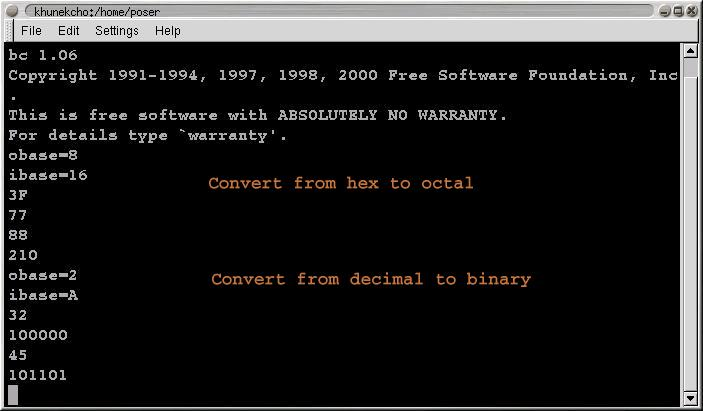# Base Conversions

Sometimes it is necessary to convert among numbers in octal, hexadecimal, binary and decimal form. For example, most current character encoding charts are in hexadecimal (base 16), but many programs (not to mention us old-timers) only accept input in octal. Making such conversions by hand is tedious. There is an easy way to do this, using the Unix utility bc. bc is an interactive arbitrary precision calculator. You can use it as a plain ordinary calculator, and you can use it when you need to perform calculations involving very large numbers. (For example, if you want to know what 2 to the 60th power is, type 2^60 to bc.)

One very handy facility of this program is that you can use any input base you like and any output base. Both of these default to base 10. To change this, just assign to the variables ibase and obase respectively. For example, if you want to convert hexadecimal to octal, fire up bc and type:

obase=8
ibase=16

Then enter the hexadecimal numbers you wish to convert, one at a time, each followed by a carriage return. bc will echo each number in octal.

Similarly, if you want to convert a decimal number to binary, set:

obase=2
ibase=ANote that bc is fussy about case and insists that the letters that represent 10-15 in hexadecimal must be upper case. So you must enter 3F, not 3f.

Note also that the change in input base takes effect immediately, so if you change the input base first, when you enter the output base you must use the new base. For example, if you first set the input base to 16 and then want to change the output base to decimal, you must type:

obase=A

because A is the hexadecimal notation for decimal 10. If you were to type:

obase=10

no base conversion would take place, because you would have set both input and output bases to hexadecimal.This is because 10 in base 16 is decimal 16.

To exit bc, give the command quit or type a Control-D.